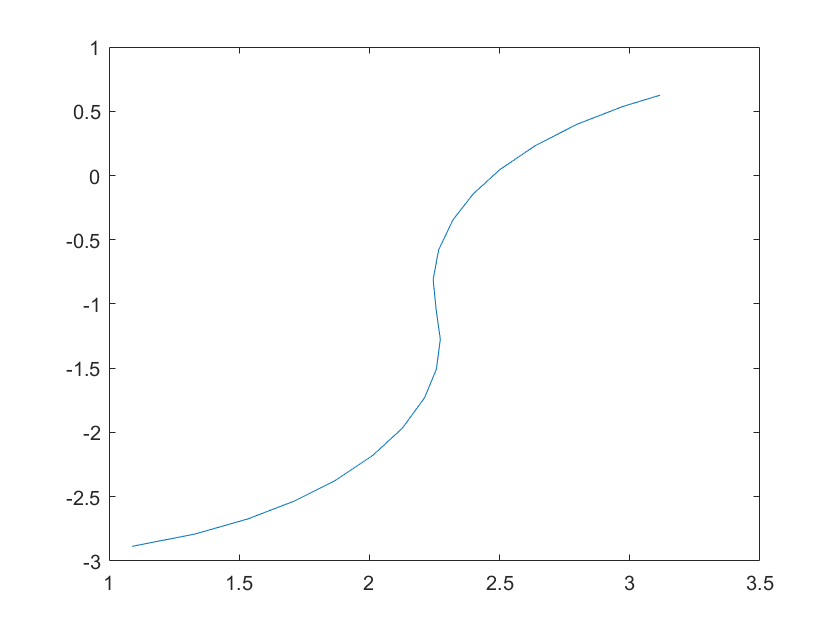# Plot Turtlebot Odometry

This example shows how to get, store, and display odometry data from a TurtleBot® as it drives in an environment.

### Connect to TurtleBot

Description of first code block

```ipaddress = '192.168.192.130'; % IP address of your robot tbot = turtlebot(ipaddress,11311); tbot.Velocity.TopicName = '/cmd_vel';```

### Get Odometry Data

Use `getOdometry` to get a single odometry point off the TurtleBot. The function returns the position and orientation as `[x y z]` coordinates and `[yaw pitch roll]` angles.

`odom = getOdometry(tbot)`
```odom = struct with fields: Position: [4.3772 1.4580 -0.0010] Orientation: [-2.2936 0.0032 7.8259e-06] ```

Send velocity commands to change the position of the robot. To note the change in position, reset the odometry first. Use `setVelocity` to drive the robot forward for 2 seconds and wait for the robot to execute this command.

```resetOdometry(tbot) setVelocity(tbot,0.5,'Time',2)```

Get the odometry after the velocity command is sent. The x-coordinate of `odom.Position` reflects the change in position. A velocity of 0.5 m/s for 2 seconds yields a displacement of 1m in the x direction as expected.

`odom = getOdometry(tbot)`
```odom = struct with fields: Position: [3.2717 0.7216 -0.0010] Orientation: [-2.6151 0.0032 -1.5861e-05] ```

### Plot Odometry Data Points

You can store odometry data to get a full trajectory of a robot path as it navigates through its environment.

Start a loop to store the odometry position and then send a velocity command. This loop runs 20 times to create a basic two-turn trajectory. Plot the trajectory.

```odomList = zeros(20,2); resetOdometry(tbot) for i = 1:20 odom = getOdometry(tbot); odomList(i,:) = [odom.Position(1) odom.Position(2)]; if i < 10 setVelocity(tbot,0.25,0.15) else setVelocity(tbot,0.25,-0.15) end pause(1); end plot(odomList(:,1),odomList(:,2))```